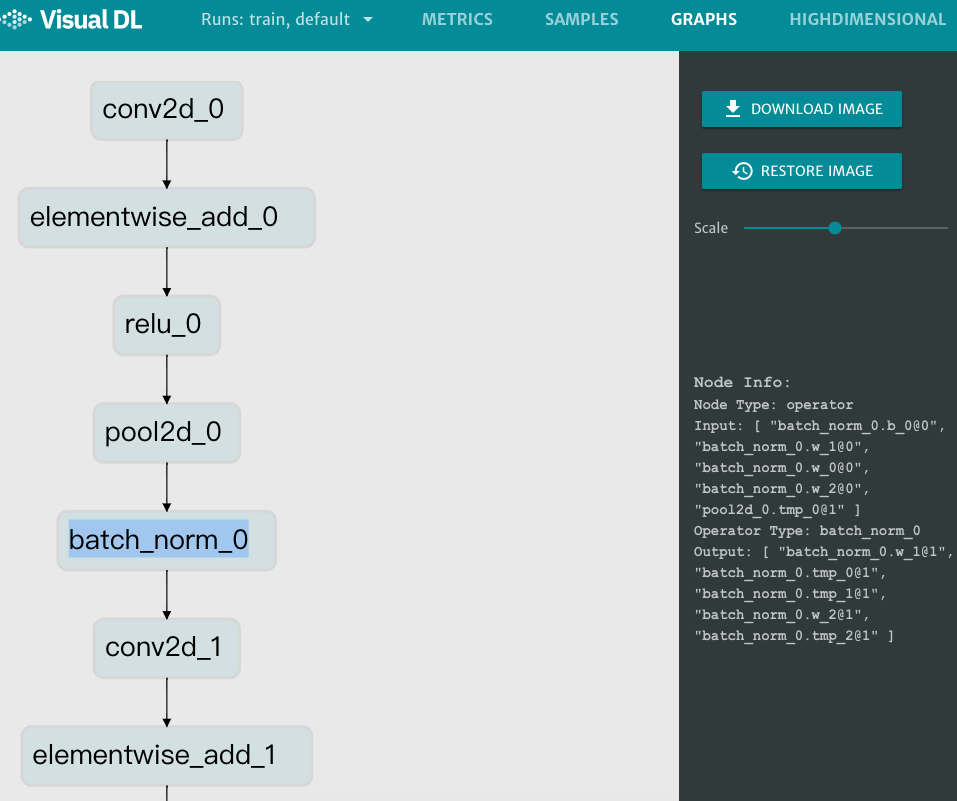# VisualDL 使用指南¶

## 概述¶

VisualDL 是一个面向深度学习任务设计的可视化工具。VisualDL 利用了丰富的图表来展示数据，用户可以更直观、清晰地查看数据的特征与变化趋势，有助于分析数据、及时发现错误，进而改进神经网络模型的设计。

scalar 折线图 动态展示损失函数值、准确率等标量数据
histogram 直方图 动态展示参数矩阵的数值分布与变化趋势，便于查看权重矩阵、偏置项、梯度等参数的变化
image 图片 显示图片，可显示输入图片和处理后的结果，便于查看中间过程的变化
text 文本 展示文本，有助于 NLP 等领域的用户进行数据分析和结果判断
audio 音频 可直接播放音频，也支持下载，有助于语音识别等领域的用户进行数据分析和结果判断
high dimensional 坐标 将高维数据映射到 2D/3D 空间来可视化嵌入，便于观察不同数据的相关性
graph 有向图 展示神经网络的模型结构

## 动态添加数据组件¶

### LogWriter -- 记录器¶

LogWriter 是一个数据记录器，在数据记录过程中，LogWriter 会周期性地将数据写入指定路径。

LogWriter 的定义为：

```class LogWriter(dir, sync_cycle)
```
:param dir : 指定日志文件的保存路径。
:param sync_cycle : 同步周期。经过 sync_cycle 次添加数据的操作，就执行一次将数据从内存写入磁盘的操作。
:return: 函数返回一个 LogWriter 对象。

```# 创建一个 LogWriter 对象 log_writer
log_writer = LogWriter("./log", sync_cycle=10)
```

LogWriter类的成员函数包括：

• `mode()`
• `scalar()`, `histogram()`, `image()`, `text()`, `audio()`, `embedding()`

```# 设定模式为 train，创建一个 scalar 组件
with log_writer.mode("train") as logger:
train_scalar = logger.scalar("acc")
# 设定模式为test，创建一个 image 组件
with log_writer.mode("test") as shower:
test_image = shower.image("conv_image", 10, 1)
```

### scalar -- 折线图组件¶

scalar 组件的输入数据类型为标量，该组件的作用是画折线图。将损失函数值、准确率等标量数据作为参数传入 scalar 组件，即可画出折线图，便于观察变化趋势。

• LogWriter 对象的成员函数 `scalar()`
```def scalar(tag, type)
```
:param tag : 标签，tag 相同的折线在同一子框，否则不同，tag 的名称中不能有 % 这个字符。
:param type : 数据类型，可选“float”, "double", "int"，默认值为 "float"。
:return: 函数返回一个 ScalarWriter 对象。
• scalar 组件的成员函数 `add_record()`
```def add_record(step, value)
```
:param step : 步进数，标记这是第几个添加的数据。
:param value : 输入数据。

```# coding=utf-8
from visualdl import LogWriter

# 创建 LogWriter 对象
log_writer = LogWriter("./log", sync_cycle=20)

# 创建 scalar 组件，模式为 train
with log_writer.mode("train") as logger:
train_acc = logger.scalar("acc")
train_loss = logger.scalar("loss")

# 创建 scalar 组件，模式设为 test， tag 设为 acc
with log_writer.mode("test") as logger:
test_acc = logger.scalar("acc")

value = [i/1000.0 for i in range(1000)]
for step in range(1000):
# 向名称为 acc 的图中添加模式为train的数据

# 向名称为 loss 的图中添加模式为train的数据
train_loss.add_record(step, 1 / (value[step] + 1))

# 向名称为 acc 的图中添加模式为test的数据
```

```visualdl --logdir ./log --host 0.0.0.0 --port 8080
```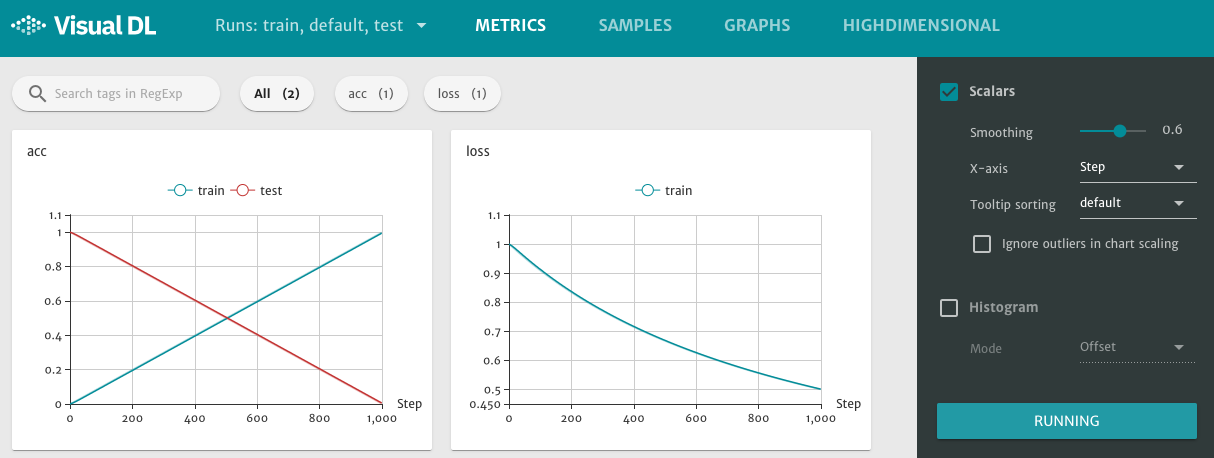VisualDL 页面的右边侧栏有各个组件的调节选项，以 scalar 组件为例：

• Smoothing : 用于调节曲线的平滑度。
• X-axis : 折线图的横坐标参数，可选 `Step`, `Relative`, `Wall Time`，分别表示横轴设为步进数、相对值、数据采集的时间。
• Tooltip sorting : 标签排序方法，可选 `default`, `descending`, `ascending`, `nearest`，分别表示默认排序、按名称降序、按名称升序、按最新更新时间排序。

VisualDL 页面的右边侧栏的最下方还有一个 `RUNNING` 按钮，此时前端定期从后端同步数据，刷新页面。点击可切换为红色的 `STOPPED`，暂停前端的数据更新。

### histogram -- 直方图组件¶

histogram 组件的作用是以直方图的形式显示输入数据的分布。在训练过程中，把一些参数（例如权重矩阵 w，偏置项 b，梯度）传给 histogram 组件，就可以查看参数分布在训练过程中的变化趋势。

• LogWriter 对象的成员函数 `histogram()`
```def histogram(tag, num_buckets, type)
```
:param tag : 标签，结合 LogWriter 指定的模式，决定输入参数显示的子框。
:param num_buckets : 直方图的柱子数量。
:param type : 数据类型，可选“float”, "double", "int"，默认值为 "float"。
:return: 函数返回一个 HistogramWriter 对象。
• histogram 组件的成员函数 `add_record()`
```def add_record(step, data)
```
:param step : 步进数，标记这是第几组添加的数据。
:param data : 输入参数， 数据类型为 list[]。

```# coding=utf-8
import numpy as np
from visualdl import LogWriter

# 创建 LogWriter 对象
log_writer = LogWriter('./log', sync_cycle=10)

# 创建 histogram 组件，模式为train
with log_writer.mode("train") as logger:
param1_histogram = logger.histogram("param1", num_buckets=100)

# 设定步数为 1 - 100
for step in range(1, 101):
# 添加的数据为随机分布，所在区间值变小
interval_start = 1 + 2 * step/100.0
interval_end = 6 - 2 * step/100.0
data = np.random.uniform(interval_start, interval_end, size=(10000))

```

```visualdl --logdir ./log --host 0.0.0.0 --port 8080
```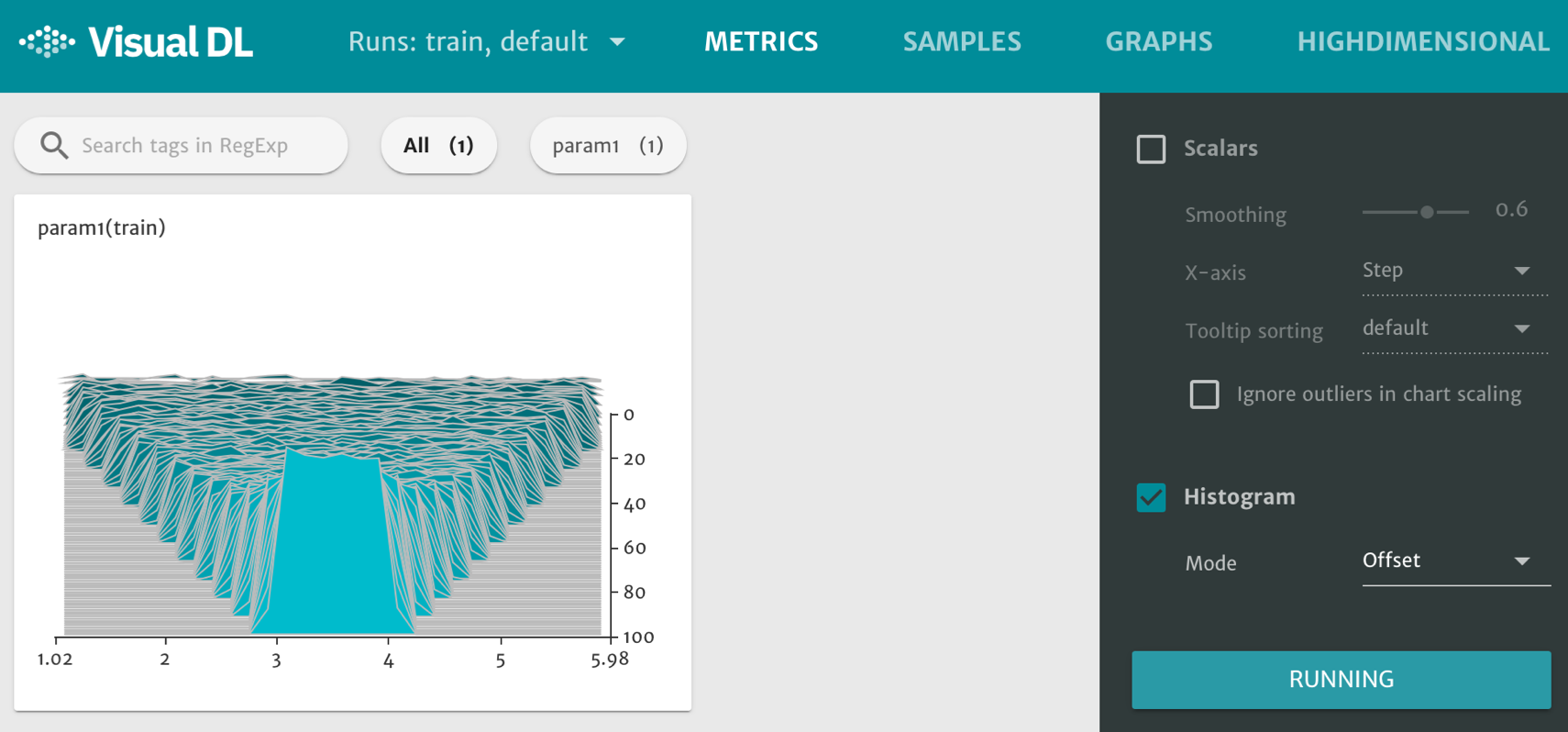### image -- 图片可视化组件¶

image 组件用于显示图片。在程序运行过程中，将图片数据传入 image 组件，就可在 VisualDL 的前端网页看到相应图片。

• LogWriter 对象的成员函数 `image()`
```def image(tag, num_samples, step_cycle)
```
:param tag : 标签，结合 set_sample() 的参数 index，决定图片显示的子框。
:param num_samples : 设置单个 step 的采样数，页面上的图片数目也等于 num_samples。
:param step_cycle : 将 step_cycle 个 step 的数据存储到日志中，默认值为 1。
:return: 函数返回一个 ImageWriter 对象。
• 开始新的采样周期 - 开辟一块内存空间，用于存放采样的数据：
```def start_sampling()
```
• 判断该图片是否应被采样，当返回值为 `-1`，表示不用采样，否则，应被采样：
```def is_sample_taken()
```
• 使用函数 `set_sample()` 添加图片数据：
```def set_sample(index, image_shape, image_data)
```
:param index : 索引号，与 tag 组合使用，决定图片显示的子框。
:param image_shape : 图片的形状，[weight, height, 通道数(RGB 为 3，灰度图为 1)]。
:param image_data : 图片的数据格式为矩阵，通常为 numpy.ndarray，经 flatten() 后变为行向量。
• 结束当前的采样周期，将已采样的数据存到磁盘，并释放这一块内存空间：
```def finish_sample()
```

```# coding=utf-8
import numpy as np
from visualdl import LogWriter
from PIL import Image

def random_crop(img):
'''
此函数用于获取图片数据 img 的 100*100 的随机分块
'''
img = Image.open(img)
w, h = img.size
random_w = np.random.randint(0, w - 100)
random_h = np.random.randint(0, h - 100)
return img.crop((random_w, random_h, random_w + 100, random_h + 100))

# 创建 LogWriter 对象
log_writer = LogWriter("./log", sync_cycle=10)

# 创建 image 组件，模式为train, 采样数设为 ns
ns = 2
with log_writer.mode("train") as logger:
input_image = logger.image(tag="test", num_samples=ns)

# 一般要设置一个变量 sample_num，用于记录当前已采样了几个 image 数据
sample_num = 0

for step in range(6):
# 设置start_sampling() 的条件，满足条件时，开始采样
if sample_num == 0:
input_image.start_sampling()

# 获取idx
idx = input_image.is_sample_taken()
# 如果 idx != -1，采样，否则跳过
if idx != -1:
# 获取图片数据
image_path = "test.jpg"
image_data = np.array(random_crop(image_path))
# 使用 set_sample() 函数添加数据
# flatten() 用于把 ndarray 由矩阵变为行向量
input_image.set_sample(idx, image_data.shape, image_data.flatten())
sample_num += 1

# 如果完成了当前轮的采样，则调用finish_sample()
if sample_num % ns == 0:
input_image.finish_sampling()
sample_num = 0
```

```visualdl --logdir ./log --host 0.0.0.0 --port 8080
```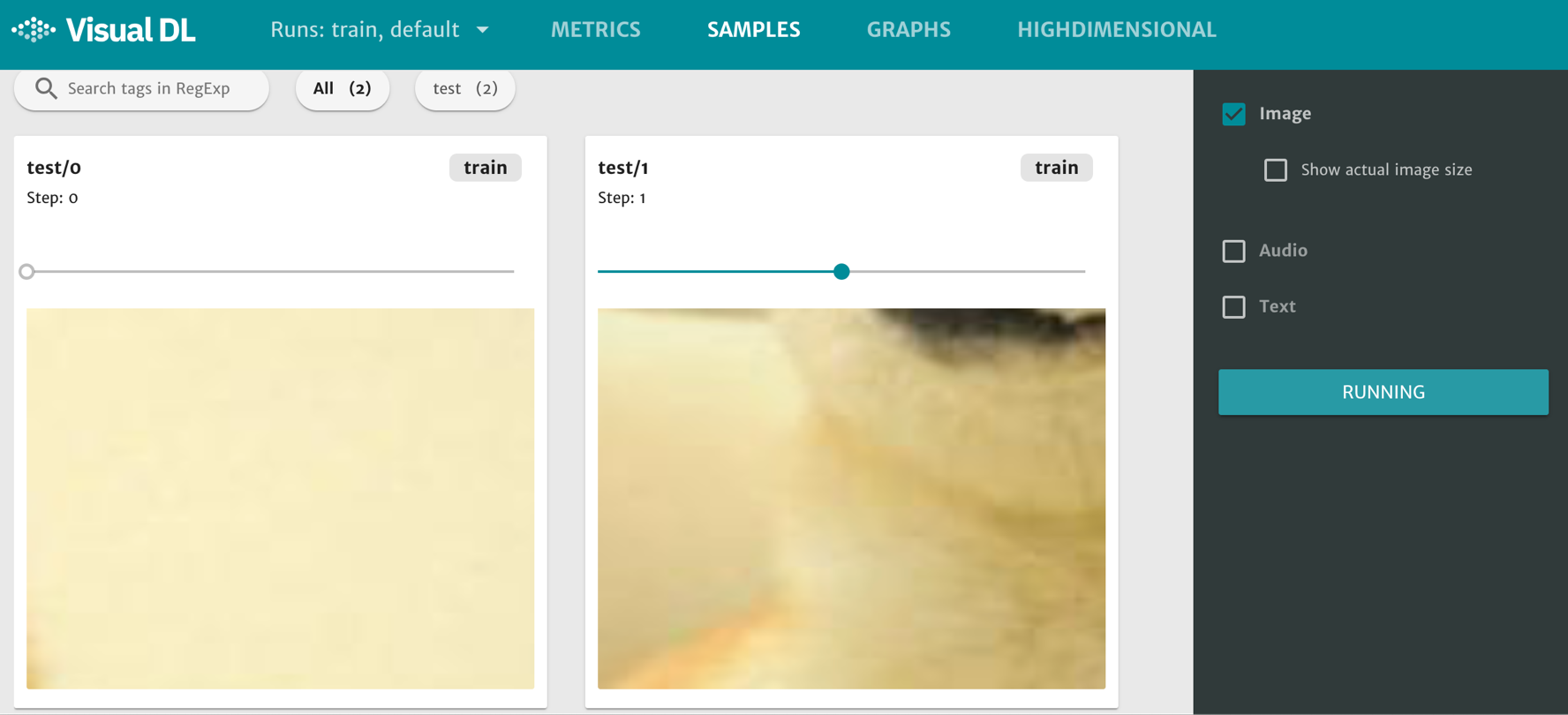### text -- 文本组件¶

text 组件用于显示文本，在程序运行过程中，将文本数据传入 text 组件，即可在 VisualDL 的前端网页中查看。

• LogWriter 对象的成员函数 `text()`
```def text(tag)
```
:param tag : 标签，结合 LogWriter 设定的模式，决定文本显示的子框。
:return: 函数返回一个 TextWriter 对象。
• text 组件的成员函数 `add_record()`
```def add_record(step, str)
```
:param step : 步进数，标记这是第几组添加的数据。
:param str : 输入文本，数据类型为 string。

```# coding=utf-8
from visualdl import LogWriter

# 创建 LogWriter 对象
log_writter = LogWriter("./log", sync_cycle=10)

# 创建 text 组件，模式为 train， 标签为 test
with log_writter.mode("train") as logger:
vdl_text_comp = logger.text(tag="test")

for i in range(1, 6):
vdl_text_comp.add_record(i, "这是第 %d 个 step 的数据。" % i)
vdl_text_comp.add_record(i, "This is data %d ." % i)
```

```visualdl --logdir ./log --host 0.0.0.0 --port 8080
```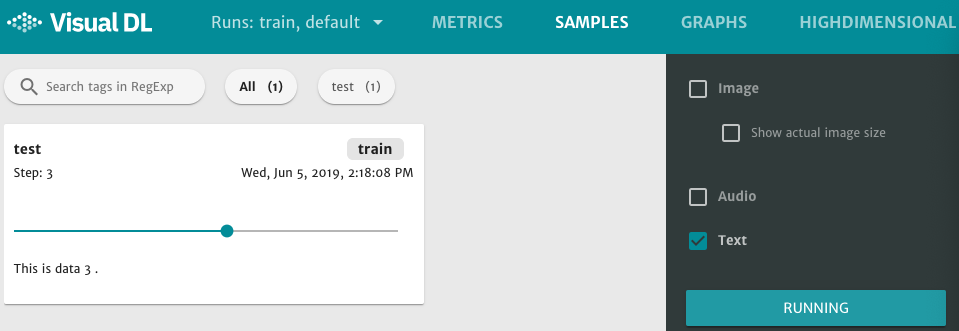### audio -- 音频播放组件¶

audio 为音频播放组件，在程序运行过程中，将音频数据传入 audio 组件，就可以在 VisualDL 的前端网页中直接播放或下载。

• LogWriter 对象的成员函数 `audio()`
```def audio(tag, num_samples, step_cycle)
```
:param tag : 标签，结合 set_sample() 的参数 index，决定音频播放的子框。
:param num_samples : 设置单个 step 的采样数，页面上的音频数目也等于 num_samples。
:param step_cycle : 将 step_cycle 个 step 的数据存储到日志中，默认值为 1。
:return: 函数返回一个 AudioWriter 对象。
• 开始新的采样周期 - 开辟一块内存空间，用于存放采样的数据：
```def start_sampling()
```
• 判断该音频是否应被采样，当返回值为 `-1`，表示不用采样，否则，应被采样：
```def is_sample_taken()
```
• 使用函数 `set_sample()` 添加音频数据：
```def set_sample(index, audio_params, audio_data)
```
:param index : 索引号，结合 tag，决定音频播放的子框。
:param audio_params : 音频的参数 [sample rate, sample width, channel]，其中 sample rate 为采样率， sample width 为每一帧采样的字节数， channel 为通道数（单声道设为1，双声道设为2，四声道设为4，以此类推）。
:param audio_data ：音频数据，音频数据的格式一般为 numpy.ndarray，经 flatten() 后变为行向量。
• 结束当前的采样周期，将已采样的数据存到磁盘，并释放这一块内存空间：
```def finish_sample()
```

```# coding=utf-8
import numpy as np
import wave
from visualdl import LogWriter

"""
读取音频数据
"""
CHUNK = 4096
f = wave.open(audio_path, "rb")
wavdata = []

while chunk:
data = np.fromstring(chunk, dtype='uint8')
wavdata.extend(data)

# 8k sample rate, 16bit frame, 1 channel
shape = [8000, 2, 1]

return shape, wavdata

# 创建一个 LogWriter 对象
log_writter = LogWriter("./log", sync_cycle=10)

# 创建 audio 组件，模式为 train
ns = 2
with log_writter.mode("train") as logger:
input_audio = logger.audio(tag="test", num_samples=ns)

# 一般要设定一个变量 audio_sample_num，用来记录当前已采样了几段 audio 数据
audio_sample_num = 0

for step in range(9):
# 设置 start_sampling() 的条件，满足条件时，开始采样
if audio_sample_num == 0:
input_audio.start_sampling()

# 获取 idx
idx = input_audio.is_sample_taken()
# 如果 idx != -1，采样，否则跳过
if idx != -1:
# 读取数据，音频文件的格式可以为 .wav .mp3 等
audio_path = "test.wav"
# 使用 set_sample()函数添加数据
input_audio.set_sample(idx, audio_shape, audio_data)
audio_sample_num += 1

# 如果完成了当前轮的采样，则调用 finish_sample()
if audio_sample_num % ns ==0:
input_audio.finish_sampling()
audio_sample_num = 0
```

```visualdl --logdir ./log --host 0.0.0.0 --port 8080
```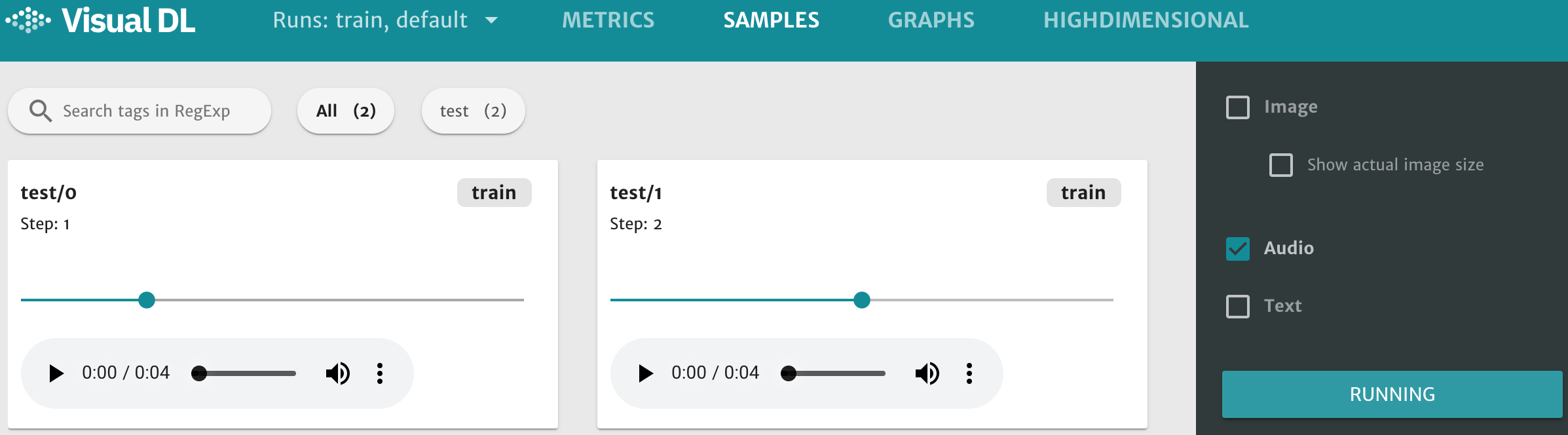### high dimensional -- 数据降维组件¶

high dimensional 组件的作用就是将数据映射到 2D/3D 空间来做可视化嵌入，这有利于了解不同数据的相关性。high dimensional 组件支持以下两种降维算法：

• PCA : Principle Component Analysis 主成分分析
• t-SNE : t-distributed stochastic neighbor embedding t-分布式随机领域嵌入

• LogWriter 对象的成员函数 `embedding()` 不需输入参数，函数返回一个 embeddingWriter 对象：
```def embedding()
```
• high dimensional 的成员函数 `add_embeddings_with_word_dict()`
```def add_embeddings_with_word_dict(data, Dict)
```
:param data : 输入数据，数据类型为 List[List(float)]。
:param Dict : 字典， 数据类型为 Dict[str, int]。

```# coding=utf-8
import numpy as np
from visualdl import LogWriter

# 创建一个 LogWriter 对象
log_writer = LogWriter("./log", sync_cycle=10)

# 创建一个 high dimensional 组件，模式设为 train
with log_writer.mode("train") as logger:
train_embedding = logger.embedding()

# 第一个参数为数据，数据类型为 List[List(float)]
hot_vectors = np.random.uniform(1, 2, size=(10, 3))
# 第二个参数为字典，数据类型为 Dict[str, int]
# 其中第一个分量为坐标点的名称, 第二个分量为该坐标对应原数据的第几行分量
word_dict = {
"label_1": 5,
"label_2": 4,
"label_3": 3,
"label_4": 2,
"label_5": 1,}

```

```visualdl --logdir ./log --host 0.0.0.0 --port 8080
```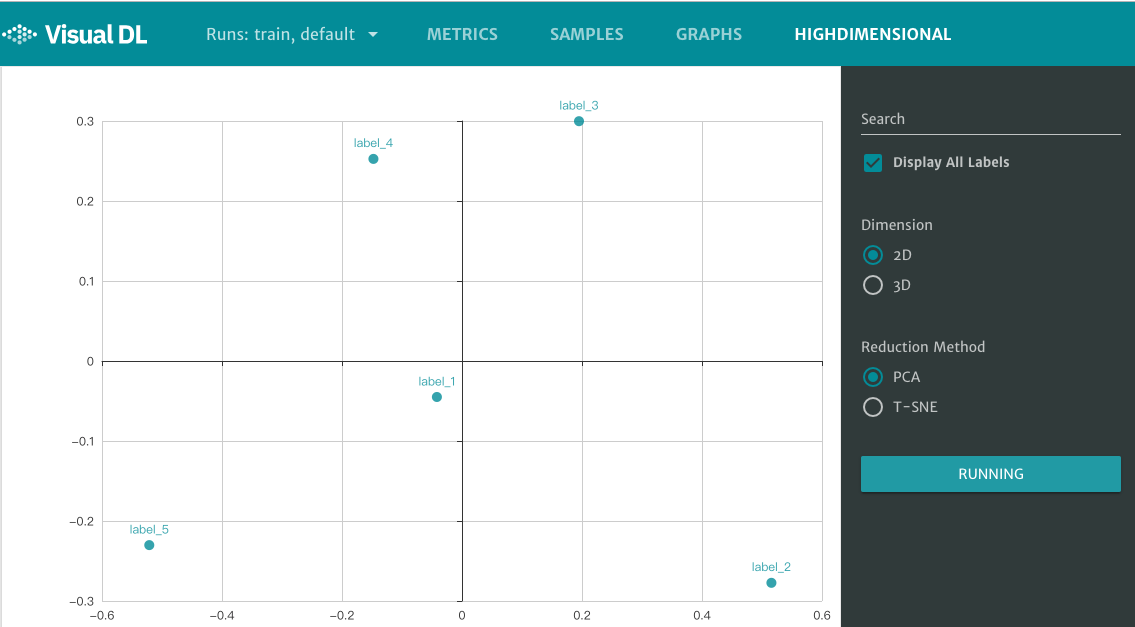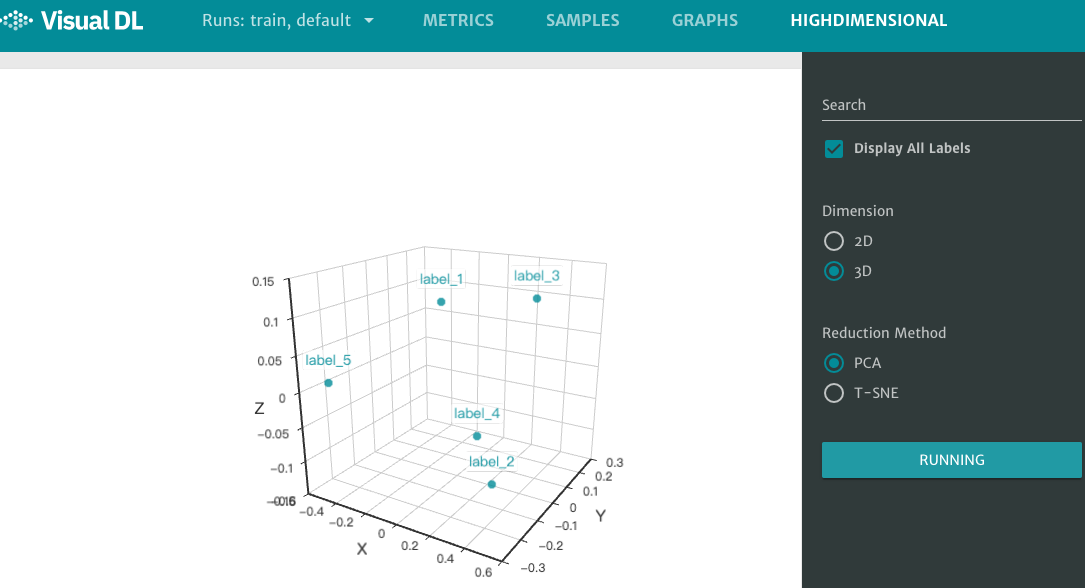## graph -- 神经网络可视化组件¶

graph 组件用于神经网络模型结构的可视化，该组件可以展示 Paddle 格式和 ONNX 格式保存的模型。graph 组件可帮助用户理解神经网络的模型结构，也有助于排查神经网络的配置错误。

```# coding=utf-8

def lenet_5(img):
'''
定义神经网络结构
'''
conv1 = fluid.nets.simple_img_conv_pool(
input=img,
filter_size=5,
num_filters=20,
pool_size=2,
pool_stride=2,
act="relu")

conv1_bn = fluid.layers.batch_norm(input=conv1)

conv2 = fluid.nets.simple_img_conv_pool(
input=conv1_bn,
filter_size=5,
num_filters=50,
pool_size=2,
pool_stride=2,
act="relu")

predition = fluid.layers.fc(input=conv2, size=10, act="softmax")
return predition

# 变量赋值
image = fluid.layers.data(name="img", shape=[1, 28, 28], dtype="float32")
predition = lenet_5(image)

place = fluid.CPUPlace()
exe = fluid.Executor(place=place)
exe.run(fluid.default_startup_program())

# 使用函数 save_inference_model() 保存 paddle 模型
fluid.io.save_inference_model(
feeded_var_names=[image.name],
target_vars=[predition],
executor=exe)
```

```visualdl --logdir ./log --host 0.0.0.0 --port 8080 --model_pb paddle_lenet_5_model
```